## Wednesday, December 23, 2015

### DTT and the pattern of comparative data

A colleague recently inquired as to how one might go about interpreting the pattern shown by a so-called disparity-through-time (DTT) plot.

A DTT plot shows the mean disparity (effectively, variance) of each subtree compared to the total disparity, and is calculated by taking a time slice at each 'event' (speciation event recorded in the reconstructed phylogeny) of the tree.

DTT plots are interpreted in a variety of ways in the literature - for instance, as evidence that variability accumulates within, rather than between, clades; or that phenotypic diversity has tended to accrue in the early rather than the later stages of a diversification.

I thought it might be nonetheless useful to conduct a 'mini' simulation study in which I examined how, in general, the accumulation of disparity among clades through time may be related to biological process.

To start with, here are a couple of small custom functions that I wrote to facilitate the analyses:

``````meanDtt<-function(D){
max.t<-max(sapply(D,function(x) max(x\$times)))
t<-seq(0,1,by=0.01)*max.t
d<-vector()
for(i in 1:length(t)){
d[i]<-0
for(j in 1:length(D)){
ii<-max(which(D[[j]]\$times<=t[i]))
d[i]<-d[i]+D[[j]]\$dtt[ii]/length(D)
}
}
obj<-list(times=t,dtt=d)
obj
}
plotDtt<-function(D,main=""){
max.d<-max(sapply(D,function(x) max(x\$dtt)))
max.t<-max(sapply(D,function(x) max(x\$times)))
plot.new()
plot.window(xlim=c(0,max.t),ylim=c(0,max.d))
for(i in 1:length(D)) lines(D[[i]]\$times,D[[i]]\$dtt,
col=rgb(0,0,1,0.1),lwd=2,
type="s")
axis(1)
axis(2)
title(xlab="time",ylab="disparity",main=main)
obj<-meanDtt(D)
lines(c(0,obj\$times),c(1,obj\$dtt),lwd=2)
}
``````

Next, we can load our packages. DTT calculation is part of the geiger package.

``````library(phytools)
``````
``````## Loading required package: ape
##
##  # ATTENTION: maps v3.0 has an updated 'world' map.        #
##  # Many country borders and names have changed since 1990. #
##  # Type '?world' or 'news(package="maps")'. See README_v3. #
``````
``````library(geiger)
``````

OK, now we can simulate some trees:

``````trees<-pbtree(n=100,scale=1,nsim=200)
trees
``````
``````## 200 phylogenetic trees
``````

OK, now let's explore the relationship between process & DTT. Our custom function, `plotDtt`, plots all the DTTs but also overlays the average DTT across simulations.

First, Brownian motion:

``````## Brownian motion
X<-lapply(trees,fastBM)
D<-mapply(dtt,trees,X,MoreArgs=list(plot=FALSE),SIMPLIFY=FALSE)
plotDtt(D,main="Brownian motion")
``````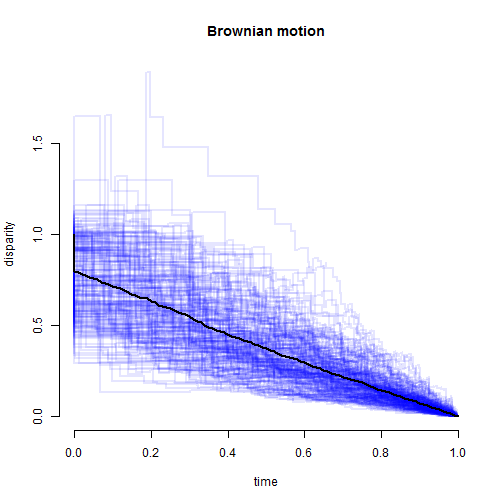Now, early-burst evolution:

``````## early burst
X<-lapply(trees,function(x) fastBM(rescale(x,"EB",a=-4)))
D<-mapply(dtt,trees,X,MoreArgs=list(plot=FALSE),SIMPLIFY=FALSE)
plotDtt(D,main="Early-Burst")
``````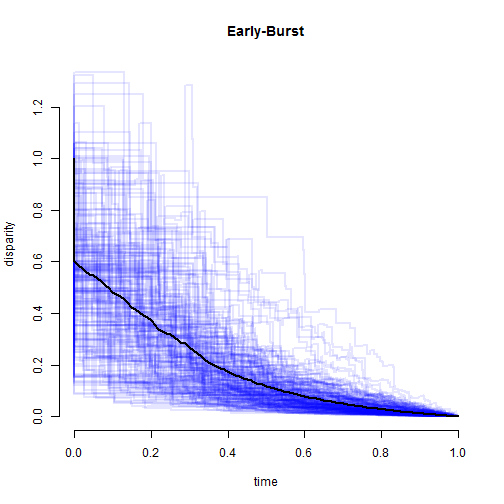Late-burst:

``````## late burst
X<-lapply(trees,function(x) fastBM(rescale(x,"EB",a=4)))
D<-mapply(dtt,trees,X,MoreArgs=list(plot=FALSE),SIMPLIFY=FALSE)
plotDtt(D,main="Late-Burst")
``````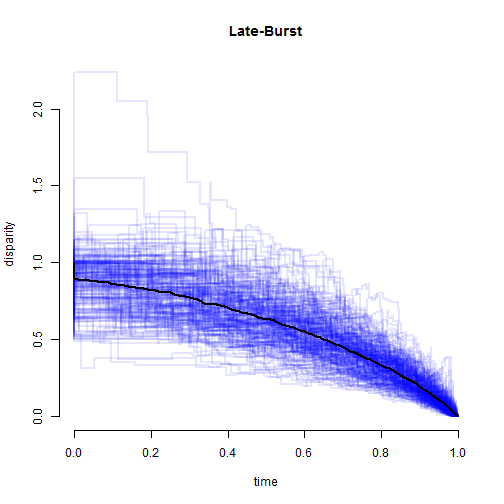Now, how about the Ornstein-Uhlenbeck model with a single optimum:

``````## OU
X<-lapply(trees,function(x) fastBM(rescale(x,"OU",alpha=4)))
D<-mapply(dtt,trees,X,MoreArgs=list(plot=FALSE),SIMPLIFY=FALSE)
plotDtt(D,main="Ornstein-Uhlenbeck")
``````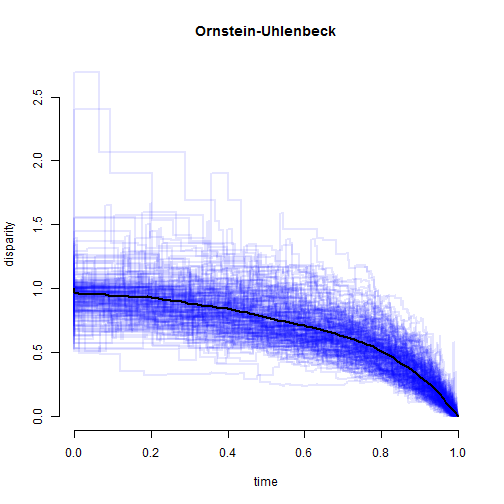In addition, here are a couple of models that we can simulate very easily, but not fit:

``````## Brownian motion with bounds
X<-lapply(trees,fastBM,bounds=c(-1,1))
D<-mapply(dtt,trees,X,MoreArgs=list(plot=FALSE),SIMPLIFY=FALSE)
plotDtt(D,main="Bounded BM [-1,1]")
``````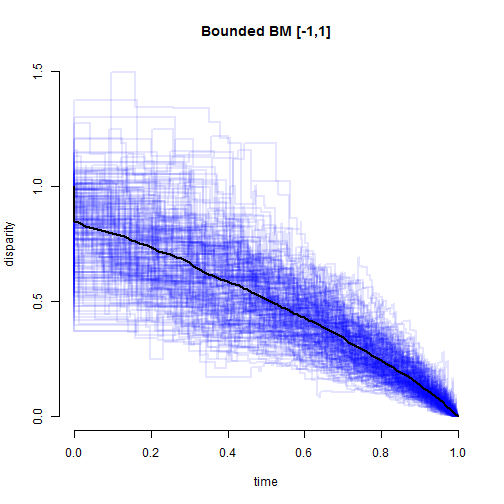``````X<-lapply(trees,fastBM,bounds=c(-0.4,0.4))
D<-mapply(dtt,trees,X,MoreArgs=list(plot=FALSE),SIMPLIFY=FALSE)
plotDtt(D,main="Bounded BM [-0.4,0.4]")
``````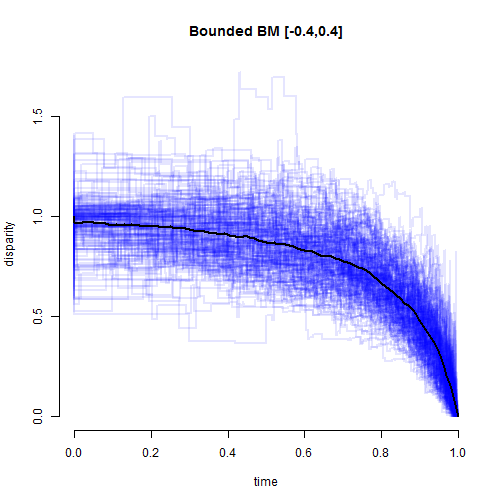``````X<-lapply(trees,fastBM,bounds=c(-0.2,0.2))
D<-mapply(dtt,trees,X,MoreArgs=list(plot=FALSE),SIMPLIFY=FALSE)
plotDtt(D,main="Bounded BM [-0.2,0.2]")
``````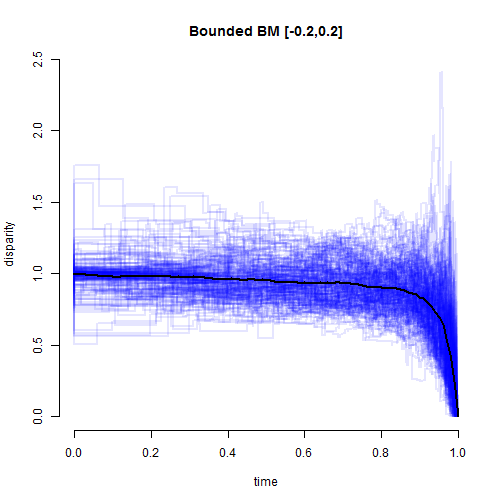``````## Brownian motion with a trend
X<-lapply(trees,fastBM,mu=4)
D<-mapply(dtt,trees,X,MoreArgs=list(plot=FALSE),SIMPLIFY=FALSE)
plotDtt(D,main="BM with a trend")
``````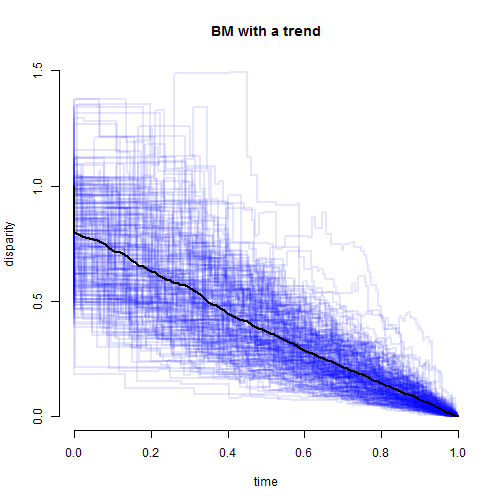Finally, it is important to keep in mine that DTT plots can be pretty substantially affected by sampling error in the estimation of species means. Here is a demo of the effect of two different levels of sampling error. I have simulated sampling variances from a exponential distribution with rate 1.0, but then multiplied by different values of a constant `k`, in which larger values imply more sampling error:

``````## measurement error
sim.error<-function(tree,k=0.1){
x<-fastBM(tree)
v<-k*setNames(rexp(n=Ntip(tree)),names(x))
sampleFrom(x,v,n=rep(1,Ntip(tree)))
}
X<-lapply(trees,sim.error,k=0.01)
D<-mapply(dtt,trees,X,MoreArgs=list(plot=FALSE),SIMPLIFY=FALSE)
plotDtt(D,"BM with sampling error (k=0.01)")
``````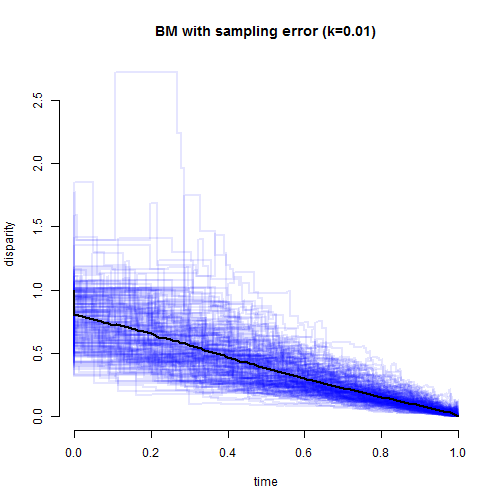``````X<-lapply(trees,sim.error,k=0.05)
D<-mapply(dtt,trees,X,MoreArgs=list(plot=FALSE),SIMPLIFY=FALSE)
plotDtt(D,"BM with sampling error (k=0.05)")
``````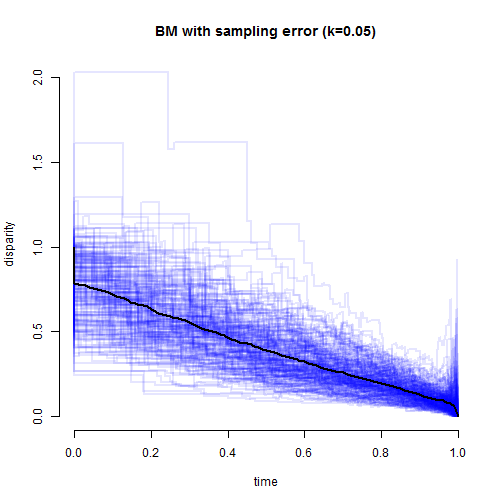``````X<-lapply(trees,sim.error,k=0.2)
D<-mapply(dtt,trees,X,MoreArgs=list(plot=FALSE),SIMPLIFY=FALSE)
plotDtt(D,"BM with sampling error (k=0.2)")
``````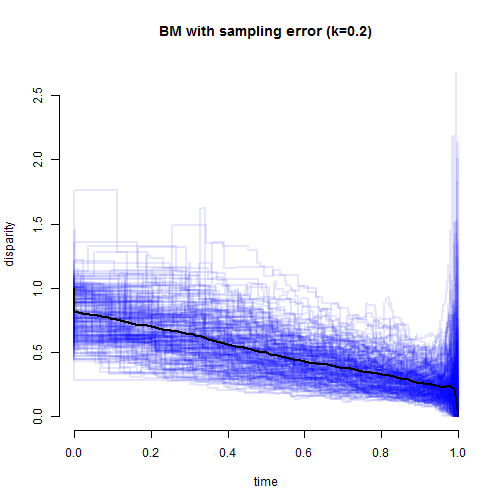That's really all I have.

1.Dear Liam, thanks for these simulations. I wonder whether "dtt" performs well also when one has multiple samples per species or whether this method is not appropriate for such datasets.
Best,
Martin

2.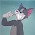This is one of the best posts i have seen in buy replica watches a long time. thanks for posting it. while there are different grades of replicas,( some better than others) it is best to read the reviews from the owners on these boards to find out replica Omega watches UK the real quality of a watch you are wanting to purchase. never listen to a seller just arbitrarily saying his product is grade 1 or AAA. he only wants your money.

3.I made my first few DTT plots and I am getting values above 1 as time approaches the present. I am having trouble finding any literature explaining this / I'm having trouble wrapping my head around the actual calculations. Do you have any input related to DTT values above 1?

Note: due to the very large amount of spam, all comments are now automatically submitted for moderation.